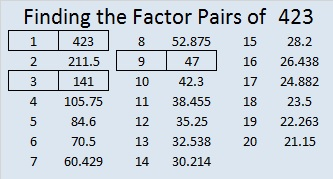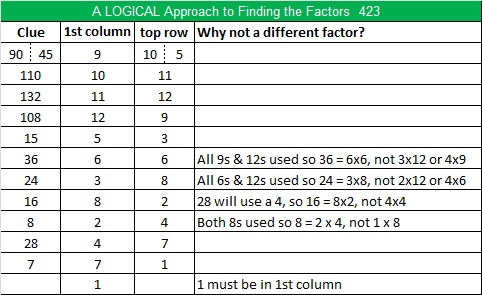# 423 and Level 4

4 + 2 + 3 = 9 therefore 423 can be evenly divided by both 3 and 9. Thus we know right away that it is a composite number whose square root can be simplified.

Also ANY natural number ending in 24 or 25 has a reducible square root, so with 423, 424, and 425 we have THREE consecutive numbers with reducible square roots. Sadly, 426 breaks the pattern so we do not have a fourth.Print the puzzles or type the factors on this excel file: 12 Factors 2015-03-09

• 423 is a composite number.
• Prime factorization: 423 = 3 x 3 x 47, which can be written 423 = (3^2) x 47
• The exponents in the prime factorization are 2 and 1. Adding one to each and multiplying we get (2 + 1)(1 + 1) = 3 x 2  = 6. Therefore 423 has exactly 6 factors.
• Factors of 423: 1, 3, 9, 47, 141, 423
• Factor pairs: 423 = 1 x 423, 3 x 141, or 9 x 47
• Taking the factor pair with the largest square number factor, we get √423 = (√9)(√47) = 3√47 ≈ 20.5670## 2 thoughts on “423 and Level 4”

1.davescarthin

Reminder – would you like tyo choose colours for your tessellation (otherwise I,m happy to do so)

2.ivasallay

Blue and yellow. Thank you for asking.

This site uses Akismet to reduce spam. Learn how your comment data is processed.